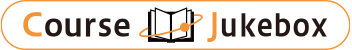### CourseDetailDegree
Master
Half
Course delivery methods
face-to-face
Subject
Computer Science
Program
School
College of Electrical Engineering & Computer Science
Department
Campus
Main Campus
Classroom
Course Offering Year
Course Offering Month
September - January
Weekday and Period
Wednesday 7,8,9
Capacity
25
Credits
3
Language
English
Course Number
EE5043

### Computer Aided Analysis & Optimization of Integrated Circuit National Taiwan University

#### Course Overview

Introduction 1. Introduction to SOC VLSI interconnect design and analysis 2. SOC design challenges and potential solutions 3. Power and signal integrity analysis and optimization Interconnect modeling and simulation 4. Formulation of circuit equation 5. Solution of linear equations 6. Interconnect delay models 7. Laplace transformation and analysis 8. Transient simulation 9. Model order reduction 10. Interconnect modeling I: capacitance extraction 11. Interconnect modeling II: inductance extraction 12. Iterative solution of linear equations & application Nonlinear circuit simulation 13. Solution of non-linear equations 14. Transient analysis of nonlinear dynamic circuits 15. Consistency, stability, convergence, local truncation error Circuit optimization 16. Mathematical programming I: linear programming 17. Mathematical programming II: nonlinear programming 18. Mathematical programming III: geometric programming 19. Combinatorial optimization I: greedy algorithm 20. Combinatorial optimization II: dynamic programming

Chung-Ping Chen

#### Other information

Graduate Institute of Electrical Engineering, Graduate Institute of Biomedical Electronics and Bioinfornatics